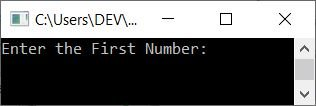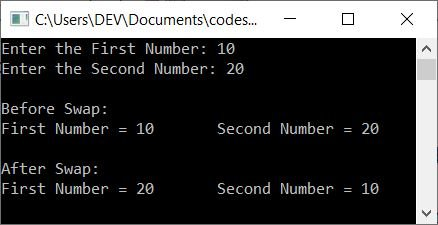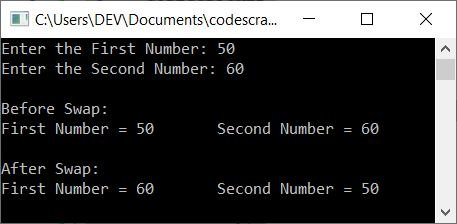# C++ Program to Swap Two Numbers

In this article, you will learn and get code to swap any two numbers entered by the user at run-time using a C++ program. Here is the list of programs available in this article:

## Swap two numbers using the third variable in C++

To swap two numbers in C++ In the programming, you have to ask the user to enter the two numbers. The two entered numbers are saved in two places. Let's call them numOne and numTwo. Now, using its variable, swap both numbers as shown in the program below:

```#include<iostream>
using namespace std;
int main()
{
int numOne, numTwo, temp;
cout<<"Enter the First Number: ";
cin>>numOne;
cout<<"Enter the Second Number: ";
cin>>numTwo;
cout<<"\nBefore Swap:\n";
cout<<"First Number = "<<numOne<<"\tSecond Number = "<<numTwo;
temp = numOne;
numOne = numTwo;
numTwo = temp;
cout<<"\n\nAfter Swap:\n";
cout<<"First Number = "<<numOne<<"\tSecond Number = "<<numTwo;
cout<<endl;
return 0;
}```

This program was built and runs under the Code::Blocks IDE. Here is its sample run:Now enter 10 as the first number and 20 as the second number.Press ENTER to swap these two numbers and print the value of both variables (that store these two values, say numOne and numTwo) before and after the swap, as shown in the snapshot given below:We've used a third variable, let's say temp, to aid in the swapping of two numbers.That is, the value of numOne gets stored in temp, and the value of numTwo gets stored in numOne. And finally, the value of temp (numOne's value) gets stored in numTwo. Swapping is accomplished in this manner.

## Swap two numbers without using a third variable in C++

To swap two numbers without using a third variable, just replace the following three lines of code (from the previous program):

```temp = numOne;
numOne = numTwo;
numTwo = temp;```

with the block of code given below:

```numOne = numOne + numTwo;
numTwo = numOne - numTwo;
numOne = numOne - numTwo;```

Also, remove the declaration of the temp variable from the program. Everything else will remain the same.

Let's suppose the user enters 10 and 20 as the first and second numbers. As a result, numOne = 10 and numTwo = 20. Now, using the three lines of code listed above. That is, after executing the following statement:

`numOne = numOne + numTwo;`

10+20 or 30 is assigned to numOne. So numOne=30. And using the following (second) statement:

`numTwo = numOne - numTwo;`

30-20 or 10 is assigned to numTwo. So numTwo=10. And using the third statement, that is:

`numOne = numOne - numTwo;`

30-10 or 20 is assigned to numOne. So numOne=20. In this way, swapping gets performed without using a third variable.

## Swap Two Numbers in C++ Using a Function (Call by Reference)

This program does the same job as the previous program. But using a user-defined function named swapFun() uses call-by-reference. Let's have a look at the program first:

```#include<iostream>
using namespace std;
void swapFun(int *, int *);
int main()
{
int numOne, numTwo;
cout<<"Enter the First Number: ";
cin>>numOne;
cout<<"Enter the Second Number: ";
cin>>numTwo;
cout<<"\nBefore Swap:\n";
cout<<"First Number = "<<numOne<<"\tSecond Number = "<<numTwo;
swapFun(&numOne, &numTwo);
cout<<"\n\nAfter Swap:\n";
cout<<"First Number = "<<numOne<<"\tSecond Number = "<<numTwo;
cout<<endl;
return 0;
}
void swapFun(int *nOne, int *nTwo)
{
int temp;
temp = *nOne;
*nOne = *nTwo;
*nTwo = temp;
}```

In the above program, inside the main() function, swapFun() gets called. It takes two arguments of the int pointer type. Therefore, we've passed the addresses of both variables, say, "numOne" and "numTwo," that hold the first and second numbers entered by the user.

Note: The * is called as the value at and & is called as the address of operator.

In this way, the address of both values (entered by the user) gets passed to the function swapFun(). Now using the * operator, we accessed the value at address and swap the numbers.

## Swap Two Numbers Using a Class in C++

This program uses classes and objects, an object-oriented feature of C++, to swap two numbers.

```#include<iostream>
using namespace std;
class CODESCRACKER
{
public:
void swapFun(int *, int *);
};
void CODESCRACKER::swapFun(int *nOne, int *nTwo)
{
int temp;
temp = *nOne;
*nOne = *nTwo;
*nTwo = temp;
}
int main()
{
int numOne, numTwo;
cout<<"Enter the First Number: ";
cin>>numOne;
cout<<"Enter the Second Number: ";
cin>>numTwo;
cout<<"\nBefore Swap:\n";
cout<<"First Number = "<<numOne<<"\tSecond Number = "<<numTwo;
CODESCRACKER c;
c.swapFun(&numOne, &numTwo);
cout<<"\n\nAfter Swap:\n";
cout<<"First Number = "<<numOne<<"\tSecond Number = "<<numTwo;
cout<<endl;
return 0;
}```

Here's a sample run with user input, with 50 as the first number and 60 as the second:An object c of type (class) CODESCRACKER is created inside the main() function. Now, through this object, we've called the member function swapFun() of the class CODESCRACKER using the dot (.) operator. Now the function operates in a similar way as a normal function does.

#### The same program in different languages

C++ Quiz

« Previous Program Next Program »